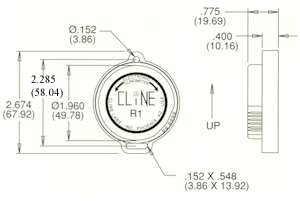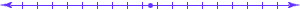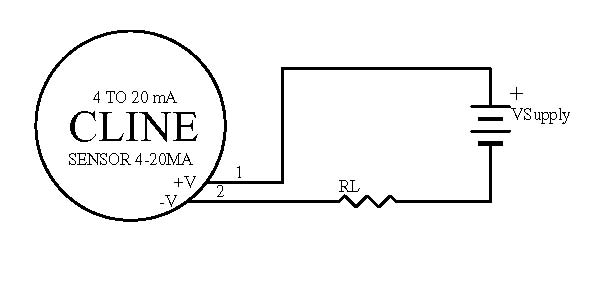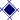4 TO 20 mA 2 Wire ANGLE MEASUREMENT SENSOR

P/N 100412-011. The voltage across the sensor must be between 8V and 36.0V.  The supply polarity is diode protected but J1.1 must be plus and J1.2 must be minus to operatate correctly.

2. The equation to determine the maximum load resistance for RL is as follows:   RL max = (Vsupply - 8) / 0.02

3. Example:  Maximum load resistance at 24 volt supply is (24 - 8) / 0.02 = 800 ohms

4. To determine the load resistance RL for a single sided 0-10 volt meter that reads the voltage across the load resistor.  10 volts / .020 mA = 500 ohm resistance.   The supply voltage VSupply must be greater than 18 volts (10 across the resistor plus 8 across the sensor) and less than 36 volts.

5. For best performance operate the sensor as follows:  Null (rotate sensor in slot) is set at 13mA,  -45 arc-degress is 5mA, and +45 arc-degrees is 21mA.   The sensor to 4-20 mA converter becomes non-linear below 5mA.General Specifications:Range:  +/- 50 degreesResolution:  .001degreeLinearity:
0 to 10 degrees is +/- 0.2 degree
10 to 45 degrees is +/-2% of Angle
45 to 50 degrees is MonotonicNull Repeatablity:  .05 degreesCross Axis Error:  <1% to 45 degreesNominal Scale Factor:  ~0.178mA/arc_degreeTypical Accuracy:  +/- 0.5% FSOTemperature
Operating is -40 to +85 degrees Celcius
Storage is -55 to +85 degrees CelciusTc of Null:  0.008 arc-degrees per  degree CelciusTc of Scale:  0.1% per degree CelciusTime Constant:  0.3 SecFreq Response:  0.5 HertzPIN ASSIGNMENTS

Description/Function

J1.1 Supply Voltage High (in)
J1.2 Supplay Voltage Low (in)CONTACT INFORMATION:

2835 West Grovers Ave. Phoenix, AZ  85053 PHONE:  (602) 863-7092 FAX:  (602) 863-7709 E-MAIL:  clinelabs@clinelabs.comHome ] Up ]